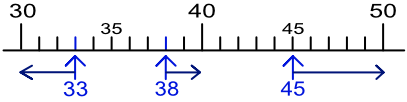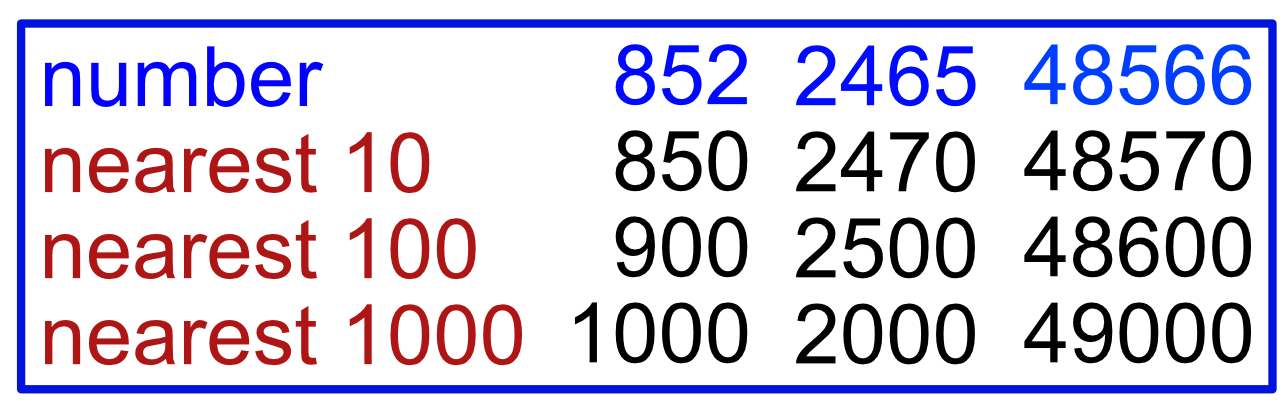Rounding to the Nearest 10, 100, 100

# Rounding to the Nearest 10, 100, 100

GCSE(F),

Rounding gives an approximate answer. For example, London to Manchester is 208 miles; but it is easier to remember it as 200 miles.

When rounding to the nearest 10, change the number to make the last digit a zero. If the last digit is 5 or more, then the value is moved up to be the next multiple of 10. If the last digit is less than 5, then the value is moved down to the previous multiple of 10.

For example, 38 is moved up in value to become 40, as 40 is the nearest 10s value. 33 is moved down in value to 30, as 30 is the nearest 10s value to 33. The number 45 is half-way between 40 and 50. Numbers on the half-way point move up in value, so 45, rounded to the nearest 10, is 50.If a number is over 100, it can still be rounded to a nearest ten. The nearest tens values to 3467 are 3460 and 3470: as the last digit is a 7, it is rounded up to 3470.

Numbers can be rounded to the nearest 100. This time, the half-way point is the value 50: less than 50 will round down; 50 or greater rounds up. Therefore 456 rounds up to 500 as the "56" part is greater than 50; and 432 will round down to 400.

Numbers can be rounded to the nearest 1000: the half-way point is 500. Examining 8462 shows that the "462" part is less than 500, so 8462 rounds down to 8000.

Some examples of rounded numbers are given below:## Examples

1. A concert hall has seats for 4425 people. How many is that to the nearest 100?